# The SEVERITY Procedure

### An Example of Modeling Regression Effects

Consider a scenario in which the magnitude of the response variable might be affected by some regressor (exogenous or independent) variables. The SEVERITY procedure enables you to model the effect of such variables on the distribution of the response variable via an exponential link function. In particular, if you have k random regressor variables denoted by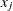(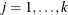), then the distribution of the response variable Y is assumed to have the form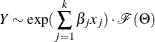where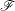denotes the distribution of Y with parameters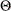and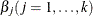denote the regression parameters (coefficients). For the effective distribution of Y to be a valid distribution from the same parametric family as, it is necessary forto have a scale parameter. The effective distribution of Y can be written as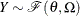wheredenotes the scale parameter and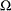denotes the set of nonscale parameters. The scaleis affected by the regressors as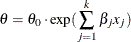where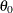denotes a base value of the scale parameter.

Given this form of the model, PROC SEVERITY allows a distribution to be a candidate for modeling regression effects only if it has an untransformed or a log-transformed scale parameter.

All the predefined distributions, except the lognormal distribution, have a direct scale parameter (that is, a parameter that is a scale parameter without any transformation). For the lognormal distribution, the parameteris a log-transformed scale parameter. This can be verified by replacingwith a parameter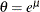, which results in the following expressions for the PDF f and the CDF F in terms ofand, respectively, where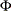denotes the CDF of the standard normal distribution: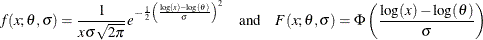With this parameterization, the PDF satisfies the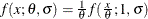condition and the CDF satisfies the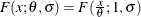condition. This makesa scale parameter. Hence,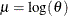is a log-transformed scale parameter and the lognormal distribution is eligible for modeling regression effects.

The following DATA step simulates a lognormal sample whose scale is decided by the values of the three regressors X1, X2, and X3 as follows: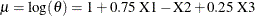/*----------- Lognormal Model with Regressors ------------*/
data test_sev3(keep=y x1-x3
label='A Lognormal Sample Affected by Regressors');
array x{*} x1-x3;
array b{4} _TEMPORARY_ (1 0.75 -1 0.25);
call streaminit(45678);
label y='Response Influenced by Regressors';
Sigma = 0.25;
do n = 1 to 100;
Mu = b(1); /* log of base value of scale */
do i = 1 to dim(x);
x(i) = rand('UNIFORM');
Mu = Mu + b(i+1) * x(i);
end;
y = exp(Mu) * rand('LOGNORMAL')**Sigma;
output;
end;
run;



The following PROC SEVERITY step fits the lognormal, Burr, and gamma distribution models to this data. The regressors are specified in the SCALEMODEL statement. The DFMIXTURE= option in the SCALEMODEL statement specifies the method of computing the CDF estimates that are used to compute the EDF-based statistics of fit.

proc severity data=test_sev3 crit=aicc print=all;
loss y;
scalemodel x1-x3 / dfmixture=full;

dist logn burr gamma;
run;



Some of the key results prepared by PROC SEVERITY are shown in Figure 23.14 through Figure 23.18. The descriptive statistics of all the variables are shown in Figure 23.14.

Figure 23.14: Summary Results for the Regression Example

The SEVERITY Procedure

Input Data Set
Name WORK.TEST_SEV3
Label A Lognormal Sample Affected by Regressors

Descriptive Statistics for y
Observations 100
Observations Used for Estimation 100
Minimum 1.17863
Maximum 6.65269
Mean 2.99859
Standard Deviation 1.12845

Descriptive Statistics for Regressors
Variable N Minimum Maximum Mean Standard
Deviation
x1 100 0.0005115 0.97971 0.51689 0.28206
x2 100 0.01883 0.99937 0.47345 0.28885
x3 100 0.00255 0.97558 0.48301 0.29709

The comparison of the fit statistics of all the models is shown in Figure 23.15. It indicates that the lognormal model is the best model according to each of the likelihood-based statistics, whereas the gamma model is the best model according to two of the three EDF-based statistics.

Figure 23.15: Comparison of Statistics of Fit for the Regression Example

All Fit Statistics
Distribution -2 Log
Likelihood
AIC AICC BIC KS AD CvM
Logn 187.49609 * 197.49609 * 198.13439 * 210.52194 * 0.68991 * 0.74299   0.11044
Burr 190.69154   202.69154   203.59476   218.32256   0.72348   0.73064   0.11332
Gamma 188.91483   198.91483   199.55313   211.94069   0.69101   0.72219 * 0.10546 *

The distribution information and the convergence results of the lognormal model are shown in Figure 23.16. The iteration history gives you a summary of how the optimizer is traversing the surface of the log-likelihood function in its attempt to reach the optimum. Both the change in the log likelihood and the maximum gradient of the objective function with respect to any of the parameters typically approach 0 if the optimizer converges.

Figure 23.16: Convergence Results for the Lognormal Model with Regressors

The SEVERITY Procedure
Logn Distribution

Distribution Information
Name Logn
Description Lognormal Distribution
Distribution Parameters 2
Regression Parameters 3

Convergence Status
Convergence criterion (GCONV=1E-8) satisfied.

Optimization Iteration History
Iter Function
Calls
-Log
Likelihood
Change Maximum
0 2 93.75285   6.16002
1 4 93.74805 -0.0048055 0.11031
2 6 93.74805 -1.5017E-6 0.00003376
3 10 93.74805 -1.421E-13 3.2667E-12

Optimization Summary
Optimization Technique Trust Region
Iterations 3
Function Calls 10
Log Likelihood -93.74805

The final parameter estimates of the lognormal model are shown in Figure 23.17. All the estimates are significantly different from 0. The estimate that is reported for the parameter Mu is the base value for the log-transformed scale parameter. Let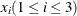denote the observed value for regressor Xi. If the lognormal distribution is chosen to model Y, then the effective value of the parametervaries with the observed values of regressors as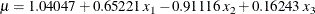These estimated coefficients are reasonably close to the population parameters (that is, within one or two standard errors).

Figure 23.17: Parameter Estimates for the Lognormal Model with Regressors

Parameter Estimates
Parameter Estimate Standard
Error
t Value Approx
Pr > |t|
Mu 1.04047 0.07614 13.66 <.0001
Sigma 0.22177 0.01609 13.78 <.0001
x1 0.65221 0.08167 7.99 <.0001
x2 -0.91116 0.07946 -11.47 <.0001
x3 0.16243 0.07782 2.09 0.0395

The estimates of the gamma distribution model, which is the best model according to a majority of the EDF-based statistics, are shown in Figure 23.18. The estimate that is reported for the parameter Theta is the base value for the scale parameter. If the gamma distribution is chosen to model Y, then the effective value of the scale parameter is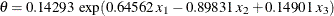.

Figure 23.18: Parameter Estimates for the Gamma Model with Regressors

Parameter Estimates
Parameter Estimate Standard
Error
t Value Approx
Pr > |t|
Theta 0.14293 0.02329 6.14 <.0001
Alpha 20.37726 2.93277 6.95 <.0001
x1 0.64562 0.08224 7.85 <.0001
x2 -0.89831 0.07962 -11.28 <.0001
x3 0.14901 0.07870 1.89 0.0613